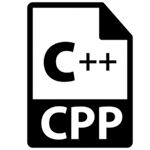# C++重载

• 方法
• 构造函数
• 索引属性

C++中的重载类型有：

• 函数重载
• 运算符重载

## C++函数重载

C++函数重载示例

```#include <iostream>
using namespace std;
class Cal {
public:
return a + b;
}
static int add(int a, int b, int c)
{
return a + b + c;
}
};
int main(void) {
Cal C;
return 0;
}```

```30
55```

## C++操作符重载

C++操作符重载示例

```#include <iostream>
using namespace std;
class Test
{
private:
int num;
public:
Test(): num(8){}
void operator ++()
{
num = num+2;
}
void Print() {
cout<<"The Count is: "<<num;
}
};
int main()
{
Test tt;
++tt;  // calling of a function "void operator ++()"
tt.Print();
return 0;
}```

`The Count is: 10`